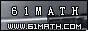Today is . WELCOME: huohai3983/44663  Home | Reg Login | All user | setHomePage | BookmarkHome | Math Test Online | Addition | Subtraction | Multiplication | Division | Time | Worksheet(JPG/PDF) | CAI | Flash Games |Recommendation articleArticle searchHome--maths--Fifth Grade--Adding Mixed Numbers with the same Denominator

Adding Mixed Numbers with the same Denominator

Adding Mixed Numbers with the same Denominator

Mixed numbers consist of an integer followed by a fraction.

How to add two mixed numbers whose fractions have the same denominator:
• Add the numerators of the two fractions
• Place that sum over the common denominator.
• If this fraction is improper (numerator larger than or equal to the denominator) then convert it to a mixed number
• Add the integer portions of the two mixed numbers
• If adding the fractional parts created a mixed number then add its integer portion to the sum.

Example: 3 2/3 + 5 2/3 =

 Add the fractional part of the mixed numbers 2/3 + 2/3 = 4/3 Convert 4/3 to a mixed number 4/3 = 1  1/3 Add the integer portions of the mixed numbers 3 + 5 = 8 Add the integer from the sum of the fractions 8 + 1 = 9 State the final answer: 9  1/3

 + =

2006-11-27 19:49:01
Viewed 2534 times CloseGreatmathsites